# Computing Vegetation Health: Student Activity

### Mini Lesson

Math Background

You can do math with units just like you do it with numbers. For example, any number divided by itself is equal to 1. The same is true of units.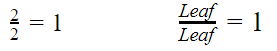Fractions made of two different units with equal magnitudes can be made into fractions equal to 1 also. For example, 1 meter = 100 cm. Since they are equal, the following fractions are equal to 1. These are called unit multipliers.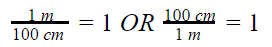When multiplying with two factors, a number and one, the answer is the factor other than one. In other words, when you multiply a number by 1, the answer is the original number. This works for fractions equal to 1.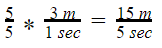Knowing this, the units can be canceled when doing calculations, just like numbers. This is shown below using several steps but can be done mentally in fewer steps.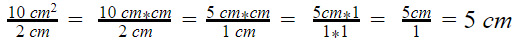This can be used with unit multipliers to convert units. The answer will have different units but it is still the same actual amount. For example, 3 m per second is equal to how many centimeters per second?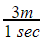needs to be converted to centimeters per second.

Use the unit multiplier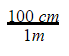and cancel units.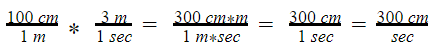This is how you determine the units for the answer to a problem. Sometimes, all the units will cancel out giving a number with no units. This often happens with ratios. NDVI, or normalized difference vegetation index, is a ratio that demonstrates this.

What is NDVI?

As can be seen through a prism, many different wavelengths make up the spectrum of sunlight. When sunlight shines on objects, certain wavelengths are absorbed and other wavelengths are reflected. The pigment in plant leaves—chlorophyll—strongly absorbs visible light for use in photosynthesis. The cell structure of the leaves, on the other hand, strongly reflects near-infrared light. The more chlorophyll, and leaves containing chlorophyll, a tree has, the more these wavelengths of light are affected. Scientists exploit this knowledge of plants' interactions with light to map the density of green vegetation across Earth's landscapes by designing satellite sensors to measure the wavelengths of red and near-infrared light that is absorbed and reflected by plants all over the world. Subtracting plants' reflectance of red light from near-infrared light and then dividing that difference by the addition of the red and near-infrared light reflected produces a value that scientists call the Normalized Difference Vegetation Index (NDVI). NDVI is the ratio of the difference of near-infrared light and red light reflectance to the sum of the near-infrared and red light reflectance.

Why is NDVI Important?

NDVI helps us monitor vegetation to:

• monitor the health of vegetation
• monitor ecosystems for disturbances
• determine where vegetation is thriving
• identify where plants are under stress

This information is important to monitor because humans rely on healthy plants for food, clothing, shelter, oxygen, absorption of carbon dioxide and medications.

How is NDVI Calculated?

Again, subtracting plants' reflectance of red light from near-infrared light and then dividing that difference by the addition of the red and near-infrared light reflected produces a value that scientists call the Normalized Difference Vegetation Index (NDVI). For example, an area that reflects 40 percent of near-infrared light and 30 percent of red light results in the following equation: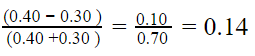The NDVI value of 0.14 indicates that vegetation in this area is very sparse. Since the numbers in the calculation are percentages expressed as decimal numbers, they are already dimensionless. The units used to calculate the percentages already canceled out.Therefore, NDVI has no unit. Rather, it is an index value in which higher values (0.4 to 0.9) show lands covered by green, leafy vegetation and lower values (0 to 0.4) show lands where there is little or no vegetation. Negative values tend to indicate the presence of water, clouds or snow. Because there is no unit, it is dimensionless.

Mini Lesson

There is an interesting video on the Honduras Ecological Forecasting page about NDVI. It is called Let’s Focus on Preservation not Deforestation. You can watch it here

Two minutes into the video, the formula for calculating NDVI is given.

1. Why is NDVI dimensionless?
2. How is NDVI used to help determine changes in the forest?
3. Calculate the following NDVI ratios.
• Reflected near infrared light 0.5, Reflectance of red-light 0.06
• Reflected near infrared light 0.4, Reflectance of red-light 0.25
4. Can NDVI be negative? Why or why not? What does it mean?

Credits/URL

NDVI: Satellites Could Help Keep Hungry Populations Fed as Climate Changes

### Teacher Note

Teachers, these mini lessons/student activities are perfect "warm up" tasks that can be used as a hook, bellringer, exit slip, etc.

1. The link to the school/institution’s teacher directory where you are employed so we can verify that you are a teacher
2. Ensure that the school email address is provided in your response as we are unable to send to personal email accounts